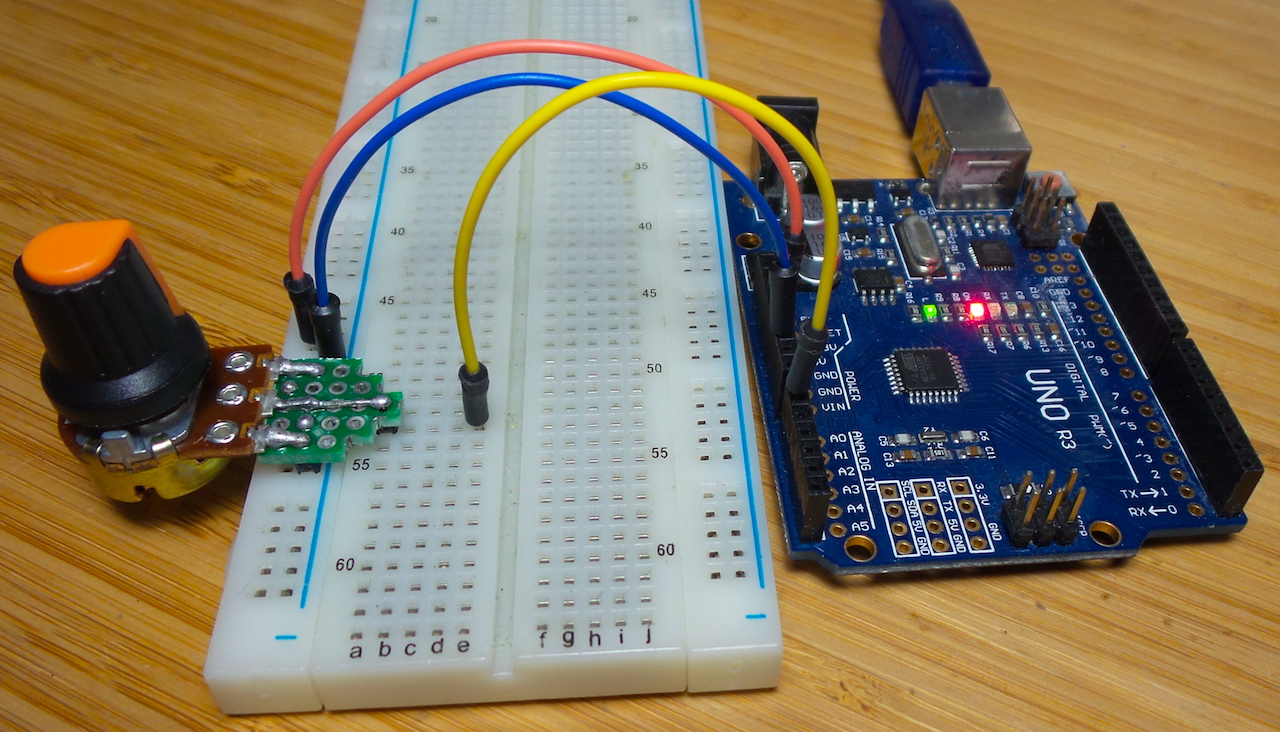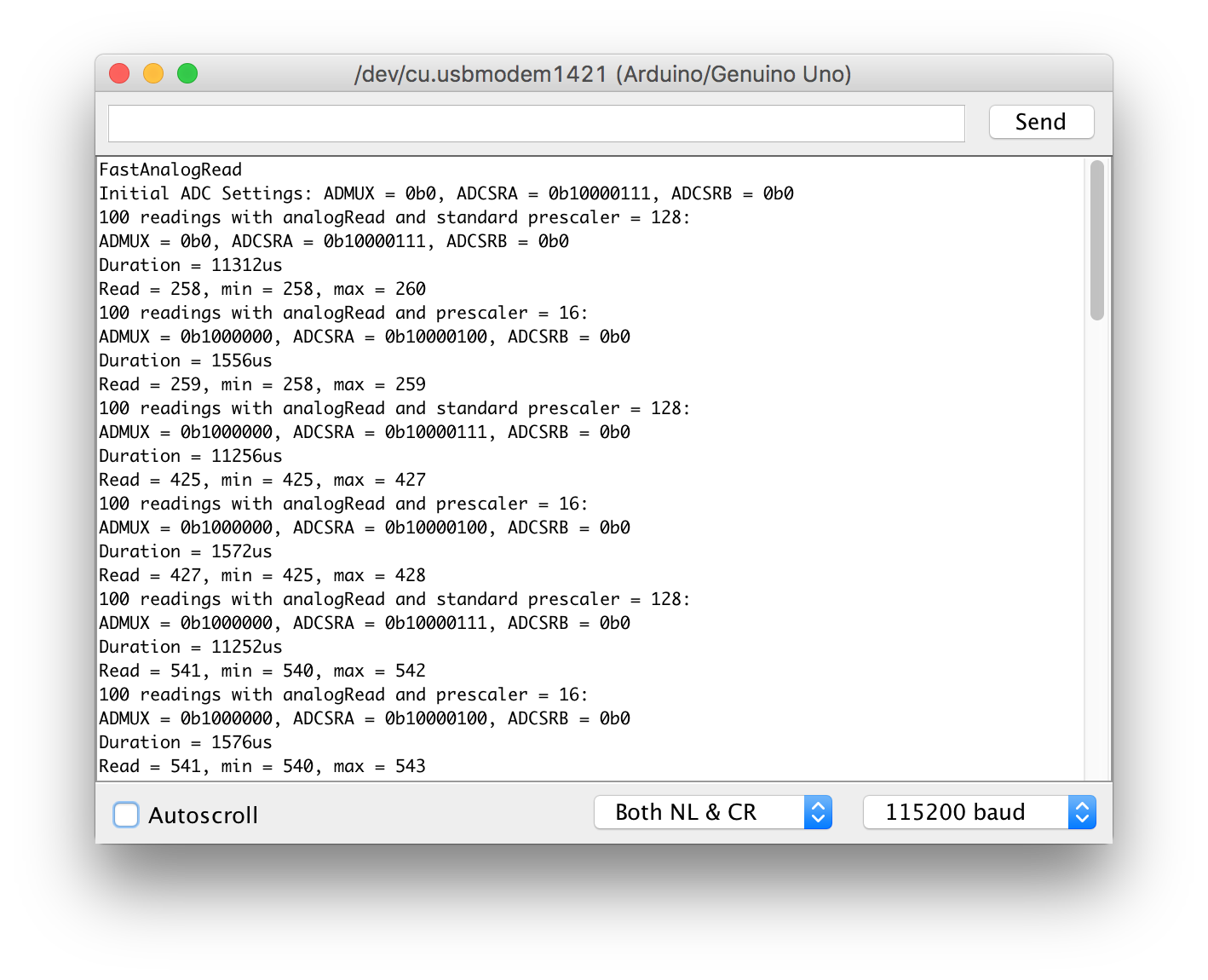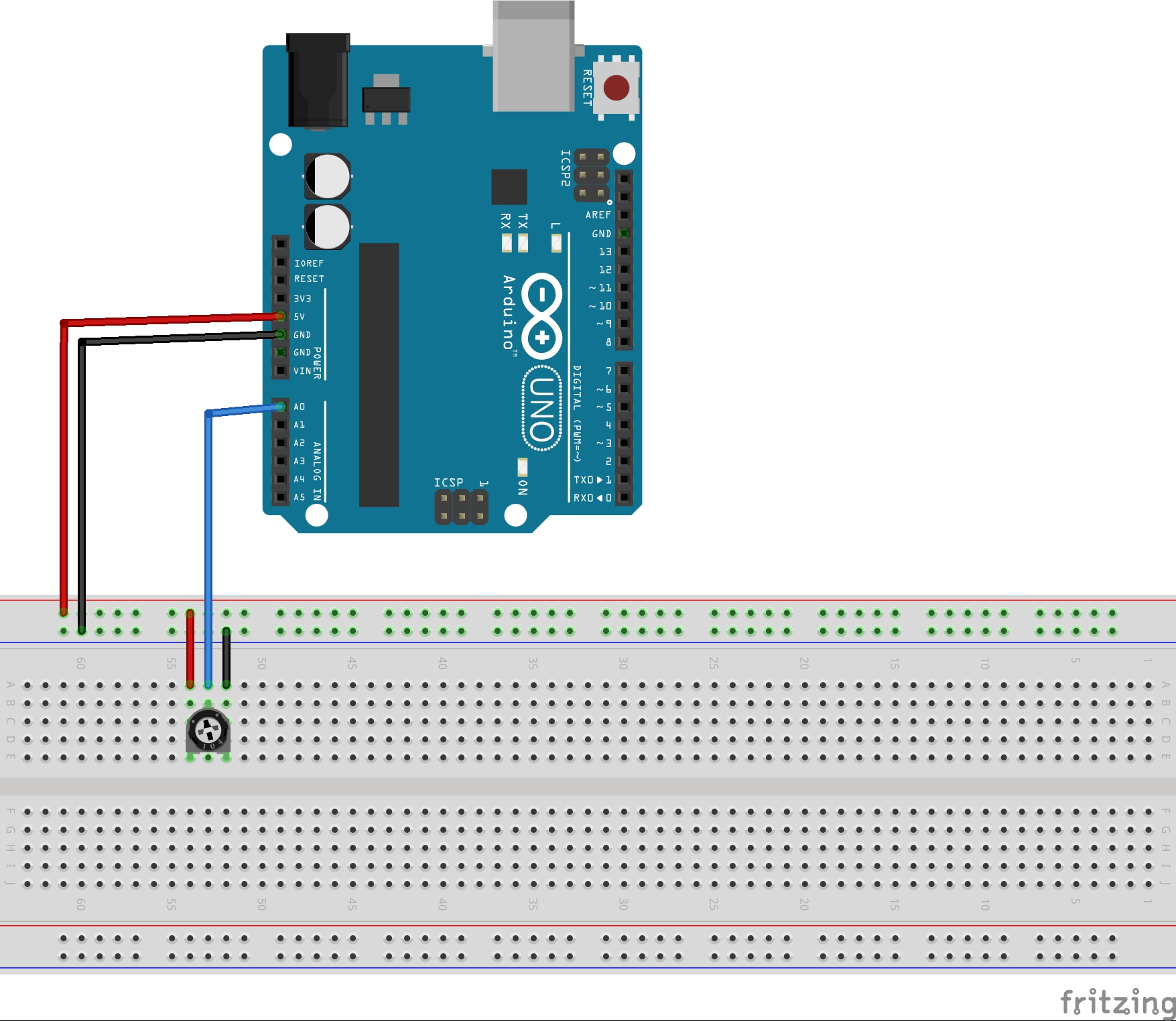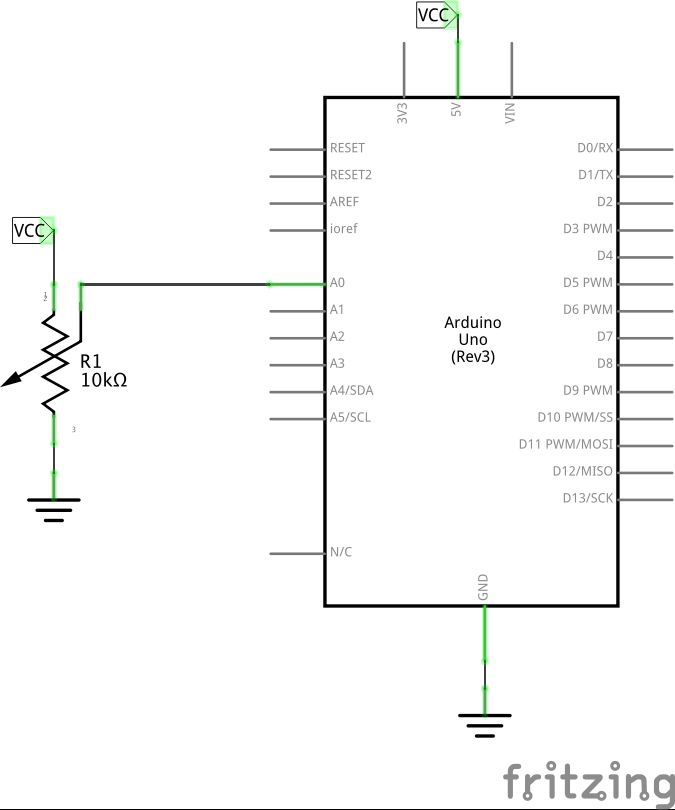### Project Notes

How to increase the Arduino ADC analog sampling rate.The ATmega328P features a 10-bit successive approximation ADC with 8-channel analog multiplexer (to support multiple analog pins).

Three registers control the behaviour of the ADC:By default, the successive approximation circuitry requires an input clock frequency between 50kHz and 200kHz to get maximum resolution. A normal conversion takes 13 ADC clock cycles.

The ADC clock may be run faster than 200kHz to get a higher sample rate, but resolution will suffer.

The data sheet quotes a sampling rate of up to 76.9kSPS, or up to 15kSPS at maximum resolution. Given that 13 clock cycles are required for a normal sample, this implies a maximum ADC frequency of up to 1MHz, or 195kHz at maximum resolution.

The ADC clock pre-scale is set with three bits of the ADCSRA register:

0 0 0 2
0 0 1 2
0 1 0 4
0 1 1 8
1 0 0 16
1 0 1 32
1 1 0 64
1 1 1 128

The initial ADPSx values are 000 i.e. pre-scale by 2. However the Arduino core adjusts the pre-scale factor during initialisation, see wiring.c. It picks that maximum pre-scale factor given the CPU frequency to keep the ADC clock within the 50kHz-200kHz band.

At 16MHz, the Arduino code sets a pre-scale of 128 (ADPSx = 111) i.e. 125kHz.

### Increasing the Sampling Rate

The FastAnalogRead.ino sketch demonstrates how to adjust the ADC pre-scaler. In the example, it is set to 16 i.e. and ADC clock frequency of 1MHz, and reads a pot on analog pin A0.

Results from a sample run:``````FastAnalogRead
Duration = 11312us
Read = 258, min = 258, max = 260
Duration = 1556us
Read = 259, min = 258, max = 259
Duration = 11256us
Read = 425, min = 425, max = 427
Duration = 1572us
Read = 427, min = 425, max = 428
Duration = 11252us
Read = 541, min = 540, max = 542
Duration = 1576us
Read = 541, min = 540, max = 543
``````

### Bandwidth

The sampling rate directly constrains the bandwidth according to the Nyquist-Shannon Theorem.

Since one conversion takes 13 ADC clock cycles:

• at default Arduino ADC frequency of 125kHz, the bandwidth is 4.8kHz
• at the increased ADC frequency of 1MHz, the bandwidth is 38.5kHz

## Construction